# Merge Sort Algorithm

Difficulty: Medium, Asked-in: Microsoft, Amazon, Goldman Sachs, Qualcomm, Bloomberg, Paytm

### Introduction to Merge Sort

Merge sort is a popular sorting algorithm that uses a divide and conquer approach to sort an array (or list) of integers (or characters or strings). Here are some excellent reasons to learn this algorithm:

• One of the fastest sorting algorithms that work in O(nlogn) time complexity.
• The best algorithm for sorting linked lists in O(nlogn) time complexity.
• An excellent algorithm to learn problem-solving using the divide and conquer approach. We can use a similar approach to solve other coding questions.
• One of the best algorithms to learn the design and analysis of recursion.
• The merge algorithm of the merge sort is an excellent idea to learn the two-pointers approach. Here both the pointers are moving in the same direction to build the partial solution. We can use a similar approach to solve other coding questions.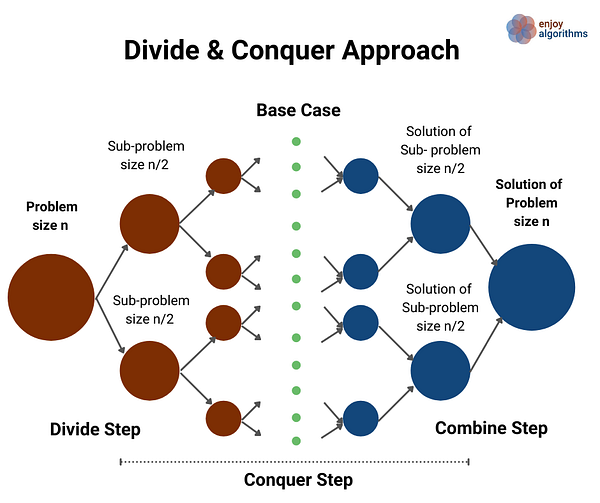### Divide and Conquer Idea of Merge Sort

Suppose we need to sort an array A[l…r] of n integers starting from index l and ending at index r. The critical question is: can we solve the sorting problem of size n using the solution of smaller sub-problems or by applying the divide and conquer strategy? Let's think!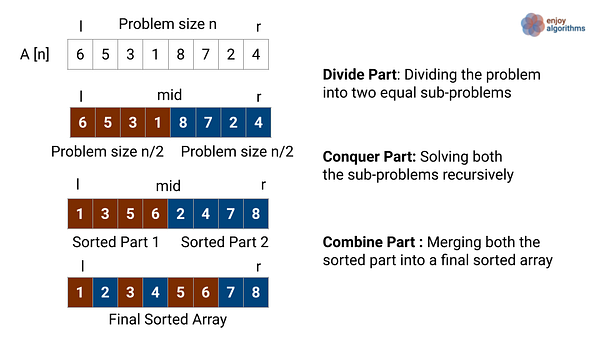If we observe the above diagram, the divide and conquer idea looks like this:

Divide Part: divide the sorting problem of size n into two different and equal sub-problems of size n/2. We can easily divide the problem by calculating the mid.

• Left subproblem: sorting A[] from l to mid
• Right subproblem: sorting A[] from mid + 1 to r

Conquer Part: Now, we solve both sub-problems recursively and sort both the smaller halves. We need not worry about the solutions to the sub-problems because recursion will do this work for us. Think!

Combine Part: we merge both the sorted halves to generate the final sorted array. In other words, we combine the solutions of both the sub-problems of size n/2 to solve the sorting problems of size n. How? Think!

### Merge Sort Implementation

Suppose the function mergeSort(int A[], int l, int r) sort the entire array A[] with left and right end as input parameters.

• Divide Part: we calculate the mid-value, mid = l + (r - l)/2.
• Conquer Part 1: we call the same function with mid as the right end and recursively sort the left part of size n/2, i.e., mergeSort(A, l, mid).
• Conquer Part 2: we call the same function with mid + 1 as the left end and recursively sort the right part of size n/2, i.e., mergeSort(A, mid + 1, r).
• Combine Part: inside the function mergeSort(), we use the function merge(A, l, mid, r) to merge both smaller sorted halves into a final sorted array.
• Base Case: If we find l == r during the recursive calls, then the sub-array has one element left, which is trivially sorted. So recursion will not go further and return from here. In other words, The sub-array of size one is the smallest version of the sorting problem for which recursion directly returns the solution.

Note: why are we not calculating mid using the formula (l + r)/2? Explore this excellent google blog to get an answer. Think!

### Merge Sort Pseudocode

``````void mergeSort(int A[], int l, int r)
{
if(l >= r)
return
int mid = l + (r - l)/2
mergeSort(A, l, mid)
mergeSort(A, mid + 1, r)
merge(A, l, mid, r)
}
``````

### The Implementation of Merging Algorithm

Solution Idea: Two Pointers Approach

After the conquer step, both left part A[l…mid] and right part A[mid+1…r] will be sorted. Now we need to combine the solution of both smaller sub-problems to build the solution of the larger problem, i.e., merging both sorted halves to create a larger sorted array. How can we do it? Let's think!

If we store the values of both sorted halves of A[] into two extra arrays of size n/2 (X[] and Y[]), then we can transform the problem into merging sorted arrays X[] and Y[] to the larger sorted array A[]. Using the sorted order property and comparing the values one by one, we can build the larger array sorted A[]. How do we implement it? Let's think!

We can use two separate pointers i and j to traverse the array X[] and Y[] from the start. We compare elements in both the arrays one by one and place a smaller value on array A[]. Another way of thinking would be: after each comparison, we add one element to the sorted output and build the partially sorted array A[]. Think! But one critical question is: can we merge these two sorted parts in place? Try to do some swapping and comparison operations to get the insight. Think!

Merging Algorithm Implementation Steps

Step 1: Memory allocation and data copy process

• We allocate the two extra arrays of size equal to the size of the left and right sorted parts i.e. size of left sorted part = mid - l +1, size of right sorted part = r - mid. Note: here we include value at mid-index in the left part.

``````int n1 = mid - l + 1
int n2 = r - mid
int X[n1], Y[n2]
``````
• Finally, we copy the left and right sorted parts of A[] into both extra arrays.

``````for (int i = 0; i < n1; i = i + 1)
X[i] = A[l + i]

for (int j = 0; j < n2; j = j + 1)
Y[j] = A[mid + 1 + j]
``````

Step 2: Now we start the merging process using two pointers loop.

• We initializes the pointers i, j, and k to traverse X[], Y[], and A[] i.e. i = 0, j = 0 and k = l. In other words, we start from the first element of both smaller arrays.
• Now we run a loop until any smaller arrays reach its end: while(i < n1 && j < n2).
• At the first step of the iteration, we compare X and Y and place the smallest value at A. Before moving forward to the second iteration, we increment the pointer k in A[] and pointer in the array containing the smaller value (maybe i or j depending on the comparison). Think!
• In a similar fashion, we move forward in all three arrays using pointers i, j, and k. At each step, we compare X[i] and Y[j], place a smaller value to A[k], and increment k by 1. Based on the comparison and position of the smaller value, we also increment the pointers i or j. if (X[i] <= Y[j]), we increment i by 1, otherwise we increment j by 1.

Note: This is a two-pointer approach of problem-solving where we build the partial solution by moving pointer i and j in the same direction.

``````int i = 0, j = 0, k = l
while (i < n1 && j < n2)
{
if (X[i] <= Y[j])
{
A[k] = X[i]
i = i + 1
}
else
{
A[k] = Y[j]
j = j + 1
}
k = k + 1
}

``````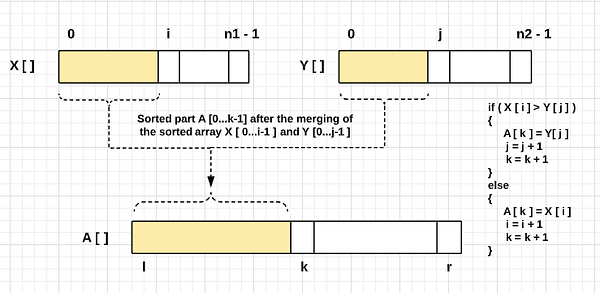Step 3: loop termination and boundary conditions

Loop will stop when any one of the two pointers reaches the end of its array (either i = n1 or j = n2). At this stage, there will be two cases of boundary conditions:

Boundary Condition 1: If we exit the loop due to condition j = n2, then we have reached the end of the array Y[] and entirely placed all the values of Y[] in A[]. But there may be some values remaining in X[] which still need to be put in the larger array A[]. The idea is: these values are greater than all the values available in A[], so we copy the remaining values of X[] in the larger array A[]. Think!

``````while (i < n1)
{
A[k] = X[i]
i = i + 1
k = k + 1
}
``````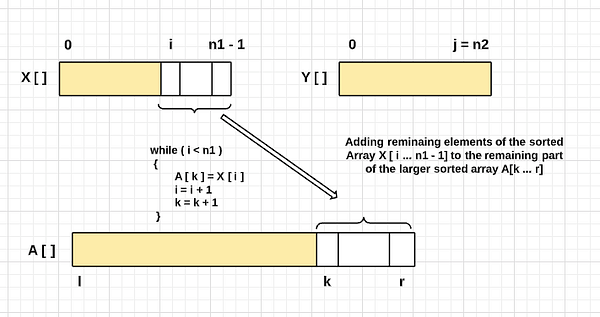Boundary Condition 2: If we exit the loop due to condition i = n1, then we have reached the end of the array X[] and entirely placed all the values of X[] in A[]. But there may be some values remaining in Y[] which still need to be put in the larger array A[]. The idea is: these values are greater than all the values available in A[], so we copy the remaining values of Y[] in the larger array A[]. Think!

``````while (j < n2)
{
A[k] = Y[j]
j = j + 1
k = k + 1
}
``````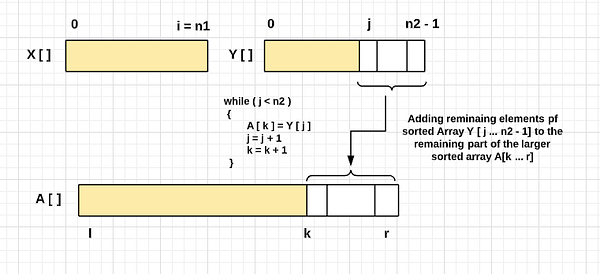Merging Algorithm Pseudocode

``````void merge(int A[], int l, int mid, int r)
{
int n1 = mid - l + 1
int n2 = r - mid
int X[n1], Y[n2]

for (int i = 0; i < n1; i = i + 1)
X[i] = A[l + i]

for (int j = 0; j < n2; j = j + 1)
Y[j] = A[mid + 1 + j]

int i = 0, j = 0, k = l
while (i < n1 && j < n2)
{
if (X[i] <= Y[j])
{
A[k] = X[i]
i = i + 1
}
else
{
A[k] = Y[j]
j = j + 1
}
k = k + 1
}

while (i < n1)
{
A[k] = X[i]
i = i + 1
k = k + 1
}

while (j < n2)
{
A[k] = Y[j]
j = j + 1
k = k + 1
}
}
``````

Time and Space Complexity Analysis of the Merging Algorithm

This is an excellent code to understand the analysis of an iterative algorithm. To understand this better, let's visualize the time complexity of each critical step and do the sum to calculate the overall time complexity.

• Memory allocation process = O(1)
• Data copy process = O(n1) + O(n2) = O(n1 + n2) = O(n)
• Merging loop in the worst case = O(n1 + n2) = O(n)
• Boundary condition 1 in the worst case = O(n1)
• Boundary condition 2 in the worst case = O(n2)
• Overall time complexity = O(1)+ O(n) + O(n) + O(n1) + O(n2) = O(n)

If we observe closely, then the merging algorithm time complexity depends on the time complexity of the merging loop where comparison, assignment, and increment are the critical operations. There could be two different perspectives to understand this analysis:

• Perspective 1: at each step of the while loop, we increment either pointer i or j. In other words, we need to access each element of both smaller arrays at least once.
• Perspective 2: at each step of the iteration, we place each element of the smaller sorted arrays to build the larger sorted array A[].

Space complexity = extra space for storing the left part + extra space for storing the right part = O(n1) + O(n2) = O(n1 + n2) = O(n)

### Merge Sort Visualization With Example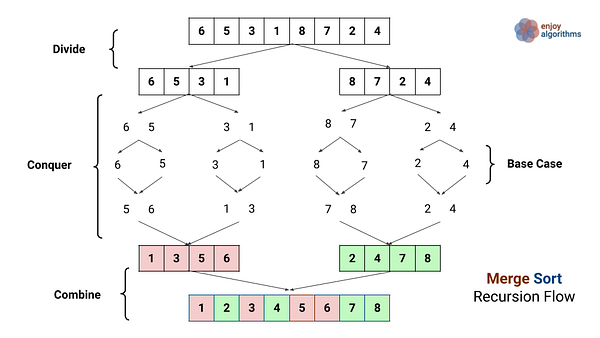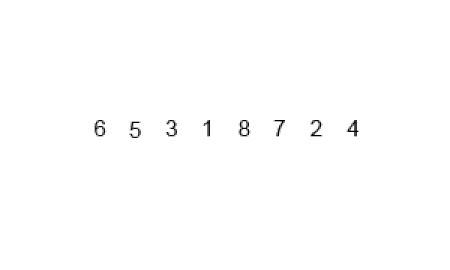### Time and Space Complexity Analysis of the Merge Sort

Let's assume that T(n) is the worst-case time complexity of merge sort for n integers. When we n >1 (merge sort on a single element takes constant time), then we can break down the time complexities as follows:

• Divide Part: the time complexity of the divide part is O(1) because calculating the middle index takes constant time.
• Conquer Part: we are recursively solving two sub-problems, each of size n/2. So the time complexity of each subproblem is T(n/2) and the overall time complexity of the conquer part is 2T(n/2).
• Combine Part: As calculated above, the worst-case time complexity of the merging process is O(n).

For calculating the T(n), we need to add the time complexities of the divide, conquer, and combine part => T(n) = O(1) + 2T(n/2) + O(n) = 2T(n/2) + O(n) = 2T(n/2) + cn

• T (n) = c, if n = 1
• T(n) = 2 T(n/2) + cn, if n > 1

Note: Merge sort function works correctly when the number of elements is not even. But to simplify the analysis, we assume that n is a power of 2 where the divide step creates the sub-problems of size exactly n/2. This assumption does not affect the order of growth of the analysis. Think!

Analysis of Merge Sort using the Recursion Tree Method

In this method, we draw a recursion tree and count the total number of operations at every level. Finally, we find the overall time complexity by doing the sum of operations count at each level.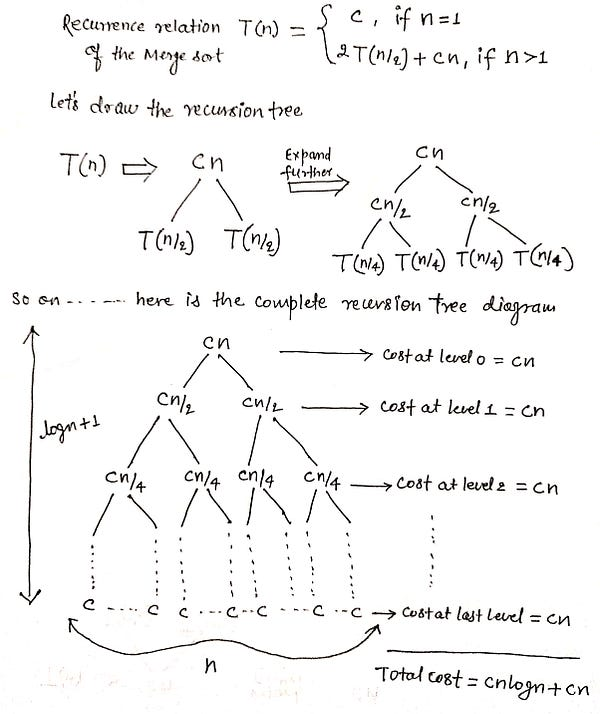Analysis of Merge Sort using the Master Theorem

Master method is a direct way to get the solution for the recurrences that can be transformed to the type T(n) = aT(n/b) + O(n^k), where a ≥ 1 and b > 1. There are the following three cases of the analysis using master theorem:

• If f(n) = O(n^k) where k < logb(a) then T(n) = O(n^logb(a))
• If f(n) = O(n^k) where k = logb(a) then T(n) = O(n^k * logn)
• If f(n) = O(n^k) where k > logb(a) then T(n) = O(n^k)

Let's compare with the merge sort recurrence

• T(n) = 2 T(n/2) + cn
• T(n) = aT(n/b) + O(n^k)

Here a = 2, b = 2 (a > 1 and b > 1)

• O(n^k) = cn = O(n¹) => k = 1
• Similarly, logb(a) = log 2(2) = 1 = k

It means, merge sort recurrence satisfy the 2nd case of the master theorem. So time complexity T(n) = O(n^k * logn) = O(n^1 * logn) = O(nlogn)

Space complexity of merge sort = space complexity of the merging process + size of recursion call stack = O(n) + O(logn) = O(n). Note: size of recursion call stack = height of the merge sort recursion tree = O(logn) (Think!)

### Critical ideas to think!

• Merge sort is a stable sorting algorithm that means that identical elements are in the same order in the input and output.
• For the smaller input, It is slower in comparison to other sorting algorithms. Even it goes through the complete process if the array is already or almost sorted.
• Merge sort is the best choice for sorting a linked list. It is relatively easy to implement a merge sort in this situation to require only O(1) extra space. On the other hand, a linked list's slow random-access performance makes some other algorithms, such as quicksort, perform poorly and others like heap sort completely impossible.
• We can parallelize the merge sort's implementation due to the divide-and-conquer method, where every smaller sub-problem is independent of each other.
• The multiway merge sort algorithm is very scalable through its high parallelization capability, which allows many processors. This makes the algorithm a viable candidate for sorting large amounts of data, such as those processed in computer clusters. Also, since memory is usually not a limiting resource in such systems, the disadvantage of space complexity of merge sort is negligible.
• We can implement merge sort iteratively without using an explicit auxiliary stack. On another side, recursive merge sort uses function call stack to store intermediate values of function parameters l and r and other information. The iterative merge sort works by considering window sizes in exponential oder, i.e., 1,2,4,8..2^n over the input array. For each window of any size k, all adjacent pairs of windows are merged into a temporary space, then put back into the array. Explore and Think! We will write a separate blog on this.

### Similar Coding Questions to Practice

Content Reference: Algorithms by CLRS

Enjoy learning, Enjoy coding, Enjoy algorithms!

Subscribe to get well-designed content on data structures and algorithms, machine learning, system design, oops, and mathematics. enjoy learning!

### We Welcome Doubts and Feedback!